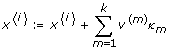# Table 1 The method ContDetApprox (left) and the main procedure sWindow (right)

ALGORITHM ContDetApprox(z, τ, b+, b-) ALGORITHM sWindow(y, t, ϵ, δ)
Input: initial state z, length τ of time interval, old boundariesInput: initial state y, times t = (t0, ..., t r ), error ϵ > 0, threshold δ > 0
Output: new boundariesOutput: probability vectors p0, ..., p r
1 for each branch i {1, ..., 2n} do 1 p0 = (1 y )T; //start with probability one in y
2    xi:= z; //z is initial state of all branches 2 for j := 1 to r do
3 end 3    τ j := t j - tj-1; //length of next time step
4 time := 0;       //define S j for construction of W j
5 Δ := step_size; //choose length of time steps 4    S j := {x | pj-1(x) >δ};
6 while time <τ do 5    numStates := min(10, size(S j ));
7    for each branch i {1, ..., 2n} do       //choose numStates random states from S j
//compare current state variables with boundaries 6    A j := rand(S j , numStates);
8       for d = 1 to n do 7    b+: -∞; b- : +∞; //initial boundaries
9          ifthen 8    for each z in A j do
10; //adjust upper bound       //run continuous determ. approximation
11          end       //on z and update boundaries
12          ifthen 9    (b+, b-) := ContDetApprox (z, τ j , b+, b-);
13; //adjust lower bound 10    end
14          end 11    Q j := generator (S j , b+, b-); //construct Q j
15       end       //construct diagonal matrix for W j (cf. Eq. (7))
16       for m := 1 to k do 12    D j := diag(S j , b+, b-);
//choose more/fewer transitions of type R m 13; //solve Eq. (7)
//depending on branch i 14 end
17          κ m := choose(α m (xi)·Δ, i);
18       end
19; //update state (cf. Eq. (11))
20    end
21    time := time + Δ;
22 end